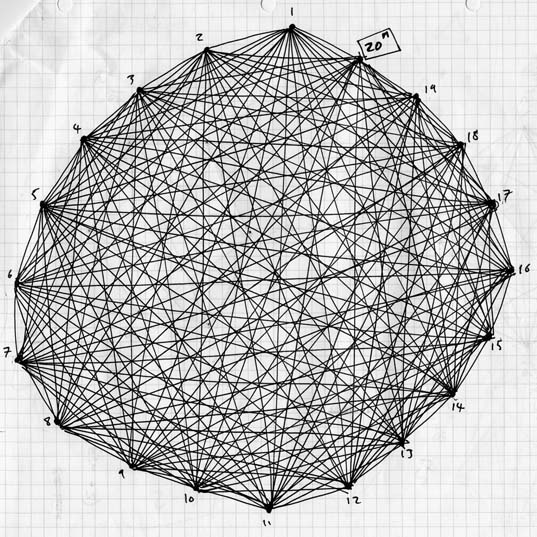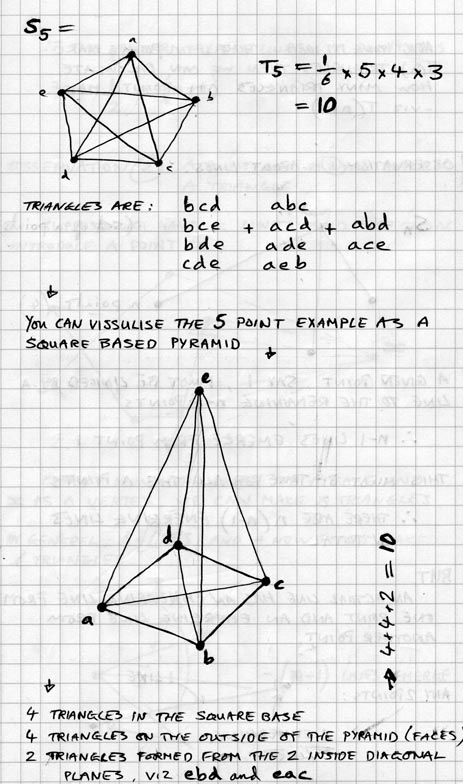THE TRIANGLE PROBLEM

IN BRIEF:
-things that grow out of idele thoughts...

a] How many triangles can I draw between a given number of dots?
b] Can I create a formula that will arnswer this question?

A project that has provided me with an opportunity to discover, illustrate and understand the problem solving inniciative of human endevour is a creative act.

In a culture where Art asks many questions and gives few answers, even with regard to it's own definition, it seems that I have been able to sneak in an answer, through the form of a-problem-solved, giving my self the opportunity of the unexpected delight of discovery.

AT LENGHT:

In thinking about and making dot-to-dots I began to wonder whether if one knew how many dots one had, could one determine, with the use of a formula, the formation of planes these dots could make. For the purposes of creating a structured mathematical investigation I decided to focus my efforts. In looking at Euclidian plane geometry, which outlines precise definitions of the geometric components such as "a point is that which has no part" and "a line is a breadthless length" (The Hutchinson Encyclopaedia, 2001:328) I decided that I would investigate the first plane shape that one could make from the smallest number of dots -the triangle. How many discrete triangles could be drawn between a given number of dots?

"I hope that you will feel drawn to problems, and experience an irresistible temptation to attempt to solve them. Good luck, and bon voyage on your mathematical journey!" (Wells, 1995:viii)

With an understanding that in mathematics "to do is to understand" and that "the simplest way to look for connections between points and lines is to do a physical experiment, and draw them" (Wells, 1995:13), I set about drawing and counting dots and triangles. As I progressed from four, to five, to six dots etc, and the diagrams became more and more complex [see n20 network drawing below], I needed to remind myself that I was counting the triangles that had a dot at each corner, rather than those triangles that formed in-between the web of lines. In insuring that I was not counting any triangles twice and therefore jeopardising my results, I began to develop a systematic approach to the drawing of the triangles that enabled me to count them as I went. It was in fact through this drawing process that I began to see past the result, into the pattern of their creation.Progressing round the circle of dots in turn, drawing all the possible new triangles that stemmed from each dot, it became apparent that from the first dot in the set, the number of triangles one could draw from this dot was two less than the total number of dots. For example, in a set of 8 dots [n=8], 6 dots can be drawn from the first dot. The number of dots that can be drawn from the second dot, moving sequentially round the circle, is also 6 and thereafter the number of triangles decreases by 1 each time one moves onto a new dot. There are however always two dots at the end of the sequence that can have no triangles drawn from them as they have been already traced out from other dots. If one were to follow a dot-to-dot that navigated one's pencil through the triangle drawing procedure [see appendix 5] and simultaneously count the number of triangles one draws from each dot, the physicality of this pattern becomes apparent.

 dots triangles After drawing the arrangements of dots and triangles, up to 23 dots [n=23], I collated my results on the following table, from which a number of things became apparent. If one takes the pattern of the digits in the unit column (of hundreds, tens, and units fame), one can see there is a symmetrical repeating pattern of 20 digits that behave as if they were flipping or rotating [see appendix 6]. This put me in mind of the arch created by the tracing of a dot, fixed on the circumference of a disc, rotating smoothly along a strait line -the cycloid. The path of the cycloid is distinctive for a number of reasons but the one which strikes home in relation to the pattern of the 20 digits is the fact that, for a moment, it is as if the curve dips below the line along which the circle rotates. What is happening at this point is that the dot on the disc is at rest: it becomes the pivot of the motion. With regard to the 20 digit repeating pattern, it is almost as if the sequence is at rest at the moments of the double 3 and 8. This provoked me to think about the behaviour of these numbers, if arranged on a disc. In remembering that "to know is to do" I proceeded to do just that; placing each of the repeating 20 digits, in the order in which they arise from the results, around a disc, allocating an equal segment of the disc to each number [see appendix 7]. From this I discovered that the difference between every opposite pairings of numbers was 5; a number that Pythagoreans believed to represent the unity of all/marriage, with 2 being man, 3 being woman, and 2+3=5. Some say though that "the Pythagoreans tossed Hippasus [a member of the Pythagorean brotherhood] overboard, drowning him, as just punishment for ruining a beautiful theory with harsh facts" (Seife, 2002:38), so one need not get too caught up in the importance of such phenomena but rather understand and appreciate the connective power of the number. n T 1 = 0 2 = 0 3 = 1 4 = 4 5 = 8 6 = 13 7 = 19 8 = 26 9 = 34 10 = 43 11 = 53 12 = 64 13 = 76 14 = 89 15 = 103 16 = 118 17 = 134 18 = 151 19 = 169 20 = 188 21 = 208 22 = 229 23 = 251

When looking at the results of the triangles investigation, it also became apparent that the difference between the numbers of consecutive results for possible triangles one can make, increasing by a value which rose by one each time: the number of triangles one can draw from 7 dots, is the sum of 6 dots plus 6, the number of triangles one can draw from 8 dots is the sum of 7 dots plus 7. This realisation enabled me to calculate sequentially and predict the value for 30 dots [n=30], and after drawing and finding the results agreed with the prediction, I could conclude an ability to predict sequentially. What I was after though was a formula that enabled me to predict at any junction, with no prior knowledge, as if sticking a pin into a map of possibilities and instantaneously be able to know my position, without reference to neighbouring values.

The patterns and relationships where staring me in the face but it was not until consultation with a number of trained and willing mathematicians that I became equipped to understand what was going on and devise a working formula for the number of triangles [T] on a single plane, between a given number of dots [n], when n>=3:

T  =  1/2 ( n (n-1))  -2

It worked! Problem solved... but do not speak too soon. I was always aware that the formula was by no means the end of the enquiry, for "the process of investigation itself enables more investigation" (Spufford, 2003:92), but was just an end, the target my desire focused upon and the momentary resolution of the process. Like a Martin Creed object or Sol LeWitt sculpture, the formula is the form that says most about the process I went through.

{{ "The game of science is, in principle, without end. He who decided one day that scientific systems do not call for any further test, and they can be regarded as finally verified, retires from the game." (Popper, 2002:32) From the initial triangle problem, I was able to develop my thinking and find a formula for predicting the number of triangles that can be made in three-dimensional space, between a given number of dots [n]: }}If one looks upon the formula as a constructed object, one is able to understand it as a mechanism for making: a production/consumption machine, values in equal values out. A machine that uses mechanisms of "the monster slag" that is mathematics (Deleuze and Guattari, 1988:24), which "enables us, it seams to me... to think about... say, water... without jumping into it" (Cage, video, 2004). In other words, in using the language of mathematics, the machine is able to achieve positions of abstraction that aids the transferability of outcomes. It is not, however, a perpetual motion machine for one needs to feed into the process in order to get anything out of it and those elements selected as inputs have a direct impact on any outcomes. That which one attributes to being n and T can vary, depending on the purposes one selects to operate the machine for. If, for example, one applies the formula to problems of scenography, the n could represent the intensity percentage off a light at a given moment and the T might be the volume at which any sound is to be set at during this same moment. This would enable a quality of the light to be directly linked to a quality of the sound for any given point. Although the practical audio-visual outcome of this may be rejected, the formula machine has provided the scenographer with an extra string to the bow of creative strategies.

A project that has provided me with an opportunity to discover, illustrate and understand the problem solving inniciative of human endevour is a creative act. In a culture where Art asks many questions and gives few answers, even with regard to it's own definition, it seems that I have been able to sneak in an answer, through the form of a-problem-solved, giving my self the opportunity of the unexpected delight of discovery.

WHAT

ZOE LAUGHLIN

HOME